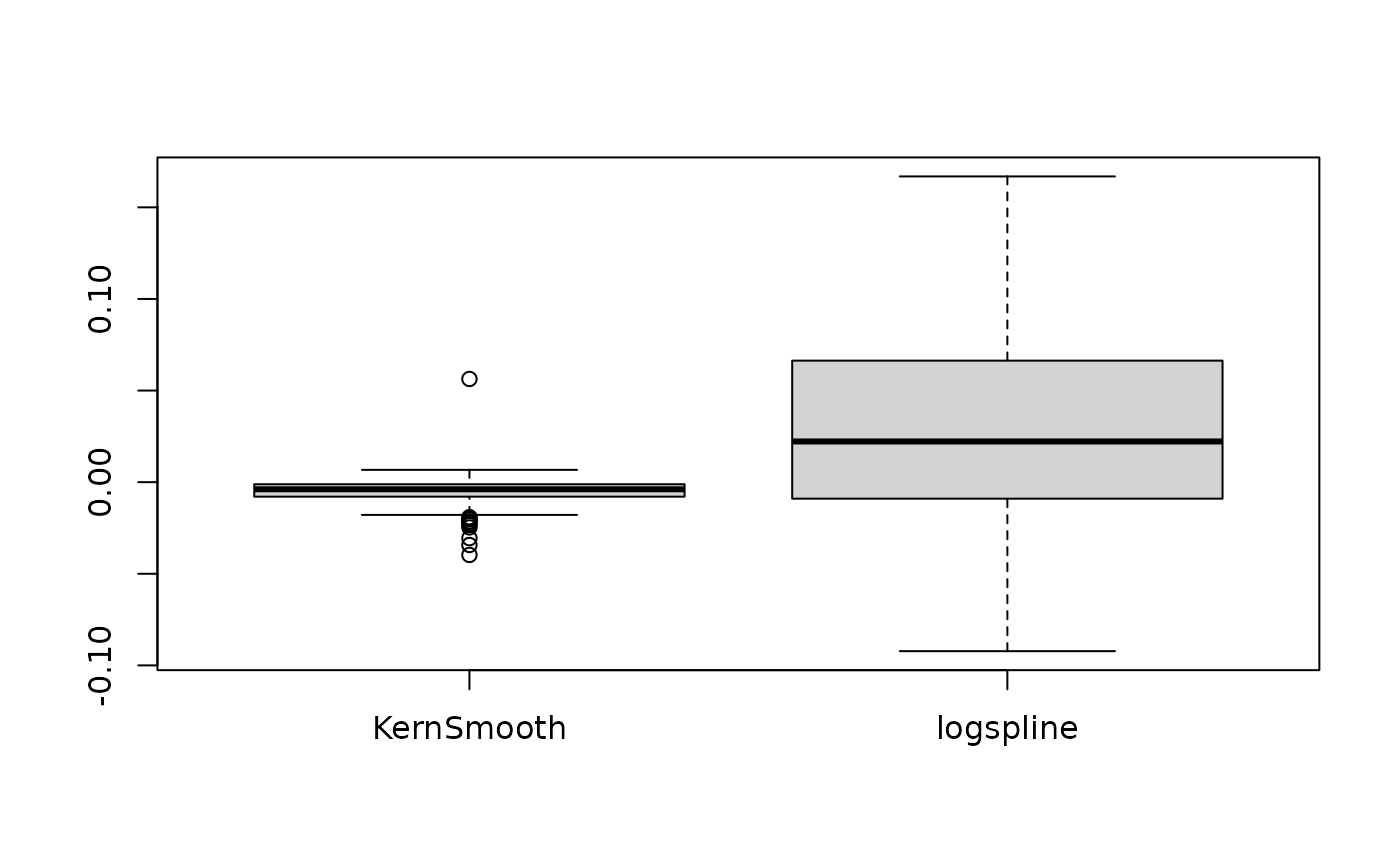Compute a Bayesian equivalent of the p-value, related to the odds that a parameter (described by its posterior distribution) has against the null hypothesis (h0) using Mills' (2014, 2017) Objective Bayesian Hypothesis Testing framework. It corresponds to the density value at 0 divided by the density at the Maximum A Posteriori (MAP).

p_map(x, precision = 2^10, method = "kernel", ...)

p_pointnull(x, precision = 2^10, method = "kernel", ...)

# S3 method for stanreg
p_map(
x,
precision = 2^10,
method = "kernel",
effects = c("fixed", "random", "all"),
parameters = NULL,
...
)

# S3 method for brmsfit
p_map(
x,
precision = 2^10,
method = "kernel",
effects = c("fixed", "random", "all"),
component = c("conditional", "zi", "zero_inflated", "all"),
parameters = NULL,
...
)

## Arguments

x Vector representing a posterior distribution, or a data frame of such vectors. Can also be a Bayesian model (stanreg, brmsfit, MCMCglmm, mcmc or bcplm) or a BayesFactor model. Number of points of density data. See the n parameter in density. Density estimation method. Can be "kernel" (default), "logspline" or "KernSmooth". Currently not used. Should results for fixed effects, random effects or both be returned? Only applies to mixed models. May be abbreviated. Regular expression pattern that describes the parameters that should be returned. Meta-parameters (like lp__ or prior_) are filtered by default, so only parameters that typically appear in the summary() are returned. Use parameters to select specific parameters for the output. Should results for all parameters, parameters for the conditional model or the zero-inflated part of the model be returned? May be abbreviated. Only applies to brms-models.

## Details

Note that this method is sensitive to the density estimation method (see the section in the examples below).

### Strengths and Limitations

Strengths: Straightforward computation. Objective property of the posterior distribution.

Limitations: Limited information favoring the null hypothesis. Relates on density approximation. Indirect relationship between mathematical definition and interpretation. Only suitable for weak / very diffused priors.

• Makowski D, Ben-Shachar MS, Chen SHA, Lüdecke D (2019) Indices of Effect Existence and Significance in the Bayesian Framework. Frontiers in Psychology 2019;10:2767. doi: 10.3389/fpsyg.2019.02767

• Mills, J. A. (2018). Objective Bayesian Precise Hypothesis Testing. University of Cincinnati.

## Examples

library(bayestestR)

p_map(rnorm(1000, 0, 1))
#> p (MAP) = 0.975p_map(rnorm(1000, 10, 1))
#> p (MAP) = 0.000
if (FALSE) {
library(rstanarm)
model <- stan_glm(mpg ~ wt + gear, data = mtcars, chains = 2, iter = 200, refresh = 0)
p_map(model)

library(emmeans)
p_map(emtrends(model, ~1, "wt"))

library(brms)
model <- brms::brm(mpg ~ wt + cyl, data = mtcars)
p_map(model)

library(BayesFactor)
bf <- ttestBF(x = rnorm(100, 1, 1))
p_map(bf)
}

# \donttest{
# ---------------------------------------
# Robustness to density estimation method
set.seed(333)
data <- data.frame()
for (iteration in 1:250) {
x <- rnorm(1000, 1, 1)
result <- data.frame(
"Kernel" = p_map(x, method = "kernel"),
"KernSmooth" = p_map(x, method = "KernSmooth"),
"logspline" = p_map(x, method = "logspline")
)
data <- rbind(data, result)
}
data$KernSmooth <- data$Kernel - data$KernSmooth data$logspline <- data$Kernel - data$logspline

summary(data$KernSmooth) #> Min. 1st Qu. Median Mean 3rd Qu. Max. #> -0.039649 -0.007867 -0.003854 -0.005315 -0.001114 0.056255 summary(data$logspline)
#>      Min.   1st Qu.    Median      Mean   3rd Qu.      Max.
#> -0.097381 -0.008781  0.023988  0.030041  0.068922  0.300639 boxplot(data[c("KernSmooth", "logspline")])# }